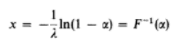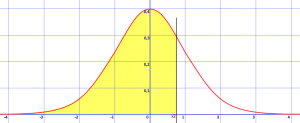# Inverse Distribution Function / Point Function / Quantile Function

Share on

Feel like "cheating" at Calculus? Check out our Practically Cheating Calculus Handbook, which gives you hundreds of easy-to-follow answers in a convenient e-book.

Contents:

## Inverse Distribution Function

The inverse distribution function (IDF) for continuous variables Fx-1(α) is the inverse of the cumulative distribution function (CDF). In other words, it’s simply the distribution function Fx(x) inverted. The CDF shows the probability a random variable X is found at a value equal to or less than a certain x. Intuitively, it’s how much area is under the curve at a certain point.

The inversion of the CDF, the IDF, gives a value for x such that:
FX(x) = Pr(X ≤ x) = s,
Where s is where random draws would fall s * 100 percent of the time (Greiner et. al, 2014).

The process sounds simple—invert the CDF— but many distributions don’t actually have simple inversions. The exponential distribution is one exception where the inverse is defined as:Good approximations are available for common functions like the normal and gamma distributions.

## Relationship Between CDF and Inverse Probability Function

The CDF gives you probabilities of a random variable X being less than or equal to some value x. The z-table is a basic example of how this works: a score found on the table shows the probability of a random variable falling to the left of the score (the “x”):Area (i.e. the probability) to the left of z = 0.8, shown in yellow, is 0.7881, or 78%.

The inverse of the CDF (i.e. the Inverse Function) tells you what value x (in this example, the z-score) would make F(x)— the normal distribution in this case— return a particular probability p. In notation, that’s:
F-1(p) = x.
To sum that all up:

• CDF = what area/probability corresponds to a known z-score?
• Inverse Function = what z-score corresponds to a known area/probability?

I used the normal distribution as an example as that’s the distribution most people seem to be familiar with. However, the concept can be applied to most distributions.

## Point Function

A point function is a way to define variable quantities that depend only on position for their values (for example, x, y, z). A point function can be scalar or vector.

## Scalar and Vector Point Function Example

A scalar point function is defined by a set of real-valued points P(x, y, z) in a scalar field; if each point has a unique scalar, then the function is a scalar point function (sometimes called scalar function of position).The distance of a any point (x, y, z) from fixed point (a, b, c) can be represented by a scalar point function.

As an example, the distance of the set of all points (x, y, z) from fixed point (a, b, c) can be represented by the following scalar point function:

f(p) = {(x a)2 + (y – b)2 + (z – c)2}½

Real-world scalar point functions can be used to represent a variety of physical systems, including (Taneja, 2010):

• The distribution of atmospheric pressure in space,
• Temperature distribution in a medium, or
• Density of a body.

A scalar point function is independent of the coordinate system: it only depends on the position of the point. For example, the temperature in a medium doesn’t depend on the choice of axes.

If, on the other hand, each point has a corresponding vector, then the function is called a vector point function (Kumar & Jaskaran, 2009). Examples of systems that can be represented by vector point functions (also called vector functions of position) include instantaneous velocity of a moving fluid, gravitational force, or electrical intensity (Taneja, 2010). If the direction of the axes is changed, the components will change, but the magnitude and direction will stay the same. For example, the velocity of a point in a moving fluid will be the same even if the axes are rotated (Urwin, 2014).

## Percent Point Function

The term “Percent Point Function” is usually used to denote a specific inverse function. For example:
“The χ2 distribution percent point function (quantile) is used with significance level α to reject the null hypothesis” (Beierle & Dekhtyar, 2015). Percent point functions exist for a wide range of distributions including the gamma distribution, Weibull distribution, triangular distribution, and many more.

## Quantile Function

The term quantile function is a synonym for the Inverse Distribution Function or Percent Point Function. It’s use is mainly restricted to software applications. For example, the SAS Quantile Function, given a specified distribution and probability, “Returns the quantile from a distribution that you specify.”

The word quantile comes from the word quantity. It refers to dividing a probability distribution into areas of equal probability.

## References

Abernathy, R. and Smith, R. (1993). Inverse Distribution Function in World Heritage Encyclopedia. Retrieved December 5, 2017 from: http://self.gutenberg.org/articles/eng/Inverse_distribution_function
Engineering Statistics Handbook (n.d). Retrieved December 5, 2017 from: http://www.itl.nist.gov/div898/handbook/eda/section3/eda362.htm
Greiner, D. et. al (2014). Advances in Evolutionary and Deterministic Methods for Design, Optimization and Control in Engineering and Sciences. Springer.
Kumar, V. & Jaskaran, D. (2009). Engineering Mathematics – II. Technical Publications.
Lewis, P. & McKenzie, E. (1988). Simulation Methodology for Statisticians, Operations Analysts, and Engineers, Volume 1. CRC Press.
Taneja, H. (2010). Advanced Engineering Mathematics. I.K. International Publishing House Pvt. Limited.
Urwin, K. (2014). Advanced Calculus and Vector Field Theory. Elsevier Science.

CITE THIS AS:
Stephanie Glen. "Inverse Distribution Function / Point Function / Quantile Function" From CalculusHowTo.com: Calculus for the rest of us! https://www.calculushowto.com/inverse-distribution-function-point-quantile/
---------------------------------------------------------------------------Need help with a homework or test question? With Chegg Study, you can get step-by-step solutions to your questions from an expert in the field. Your first 30 minutes with a Chegg tutor is free!